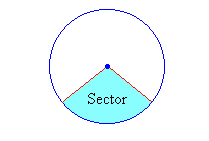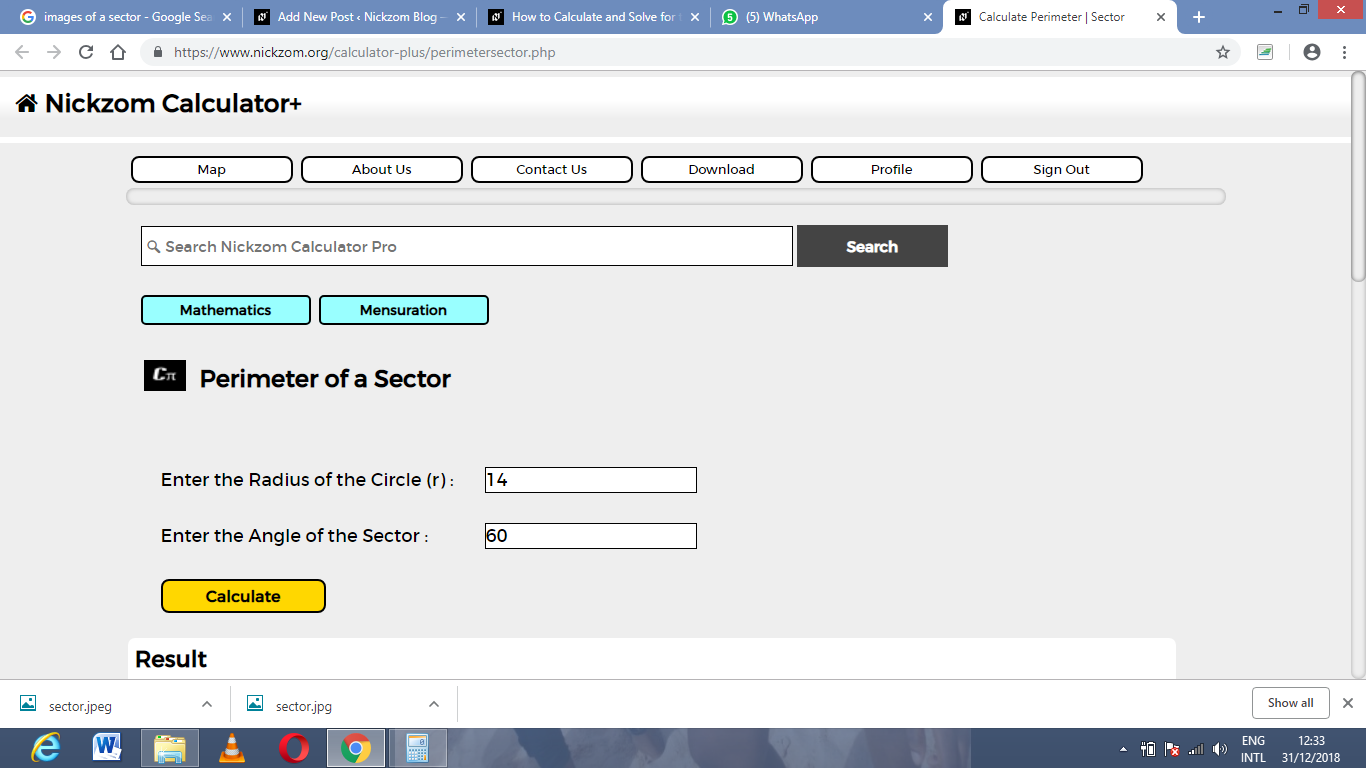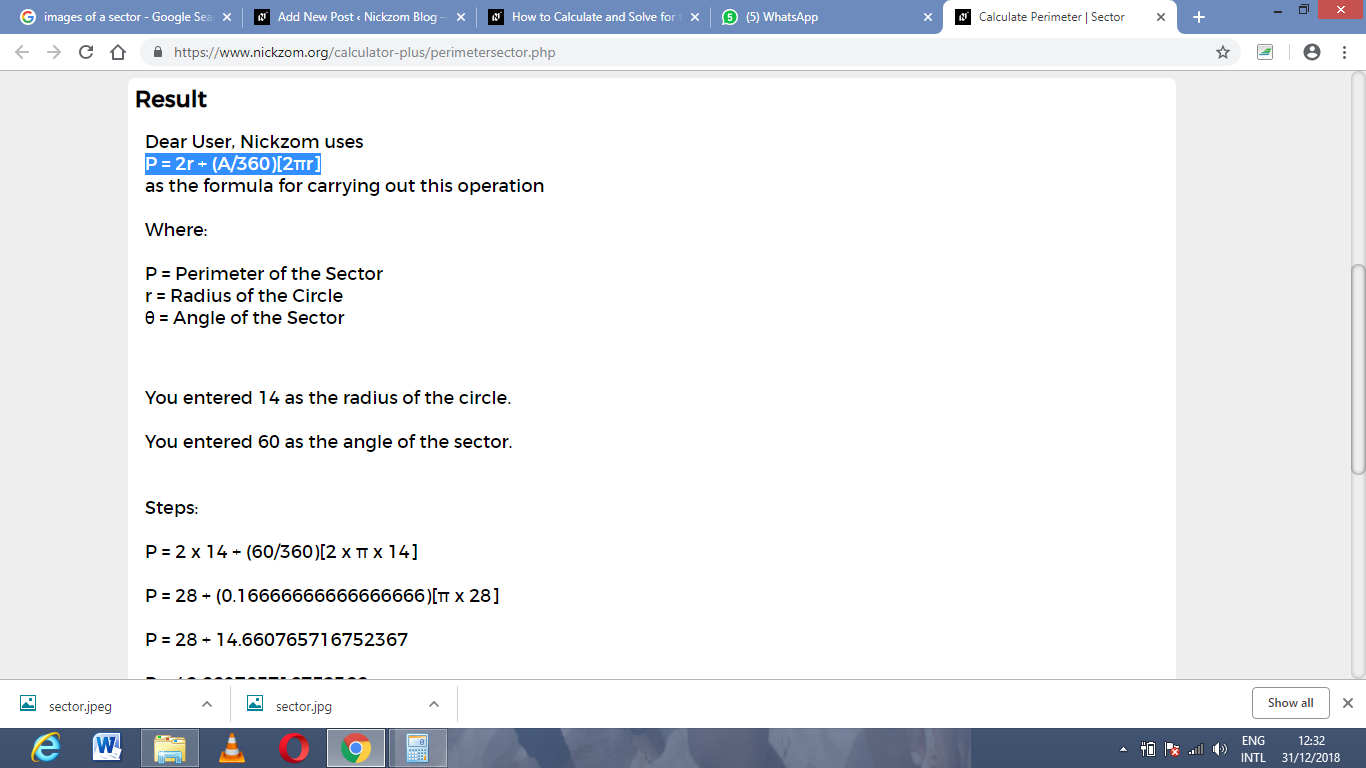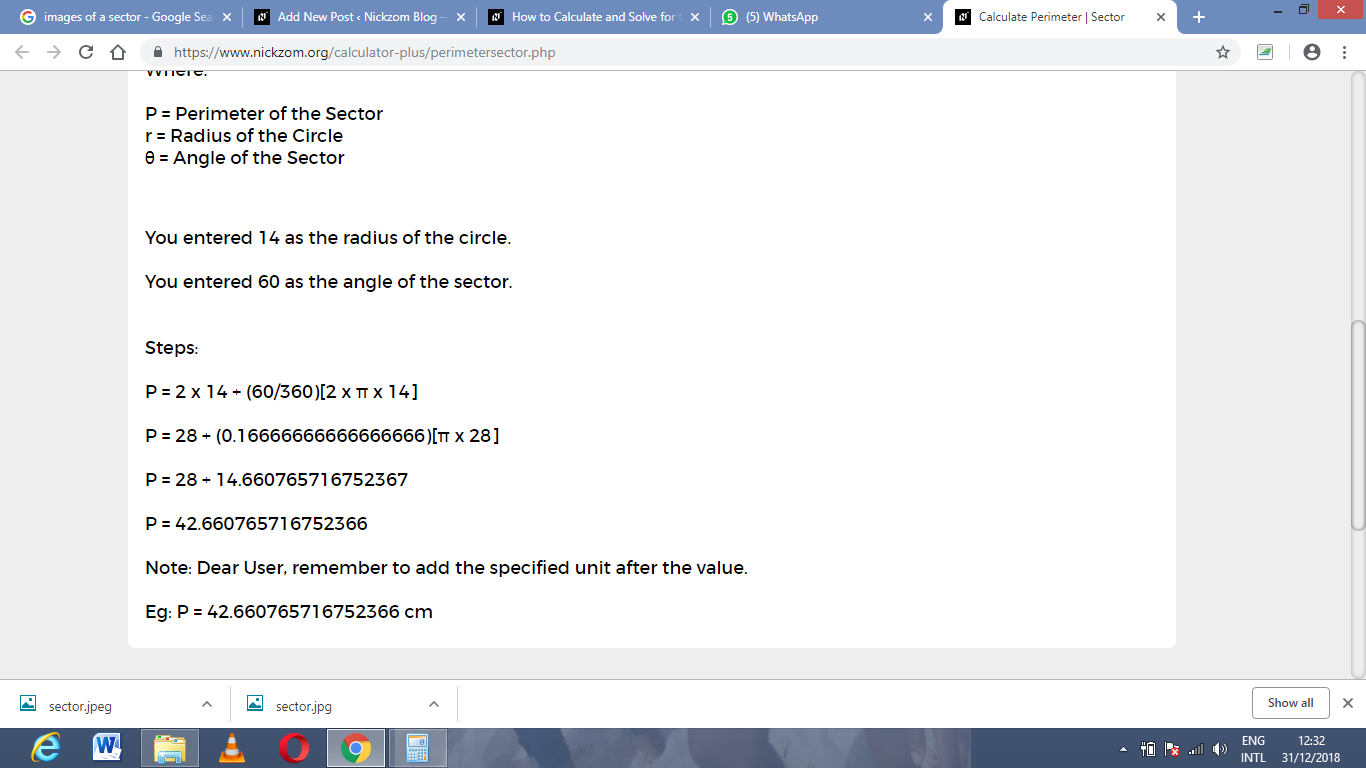# How to Calculate and Solve for the Perimeter or Circumference, Diameter, Radius and Angle of a Sector | The Calculator EncyclopediaThe image above is a sector.

To compute the Perimeter or Circumference a sector, two essential parameters is needed and this parameters are the radius of the sector (r) and the angle of the sector (θ). You can also use the diameter of the sector (d).

The formula for calculating the Perimeter or Circumference of a sector:

P = 2r + θ / 360(2πr)

Where;

P = Perimeter or Circumference
r = Radius of the sector
θ = Angle of the Sector

Let’s solve an example:
Find the perimeter or circumference of a sector when the radius of the sector is 14 cm and the angle of the sector is 60°

This implies that;

r = Radius of the sector = 14 cm
θ = Angle of the sector = 60°

P = 2r + θ / 360(2πr)
P = 2 x 14 + 60 / 360(2 x 3.142 x 14)
P = 28 + 0.1667 (87.97)
P = 28 + 14.66
P = 42.66

Therefore, the perimeter or circumference of the sector is 42.66 cm.

Calculating the Perimeter or Circumference of a Sector using Diameter and Angle of the sector.

P = d + θ / 360(πd)

θ = Angle of the sector
d = Diameter of the sector

Let’s solve an example;
Find the perimeter or circumference of a sector when the diameter of the sector is 20 cm and the angle of the sector is 80°.

This implies that;

d = Diameter of the sector = 20 cm
θ = Angle of the sector = 80°

P = d + θ / 360(πd)
P = 20 + 80 / 360(3.142 x 20)
P = 20 + 0.22 (62.84)
P = 20 + 13.82
P = 33.82

Therefore, the perimeter or circumference of the sector using diameter is 33.82 cm.

How to Calculate Diameter of a Sector when Angle of the Sector and Perimeter or Circumference of the Sector is Given

d = P / [1 + θπ / 360]

Where;

P = Perimeter or Circumference of the Sector
θ = Angle of the Sector

Let’s solve an example;
Given that the perimeter or circumference of a sector is 50 cm with an angle of 120°, Find the diameter of the sector?

This implies that;

P = Perimeter or Circumference of the sector = 50 cm
θ = Angle of the Sector = 120°

d = P / [1 + θπ / 360]
d = 50 / [1 + 120 x 3.142 / 360]
d = 50 / [1 + 377.04 / 360]
d = 50 / [1 + 1.047]
d = 50 / 2.047
d = 24.4

Therefore, the diameter of the sector is 24.4 cm.

How to Calculate Radius of a Sector when Angle of the Sector and Perimeter or Circumference of the Sector is Given

r = P / [2 + 2θπ / 360]

Where;

P = Perimeter or Circumference of the Sector
θ = Angle of the Sector

Let’s solve an example;
Given that the perimeter or circumference of a sector is 100 cm with an angle of 160°, Find the diameter of the sector?

This implies that;

P = Perimeter or Circumference of the sector = 100 cm
θ = Angle of the Sector = 160°

r = P / [2 + 2θπ / 360]
r = 100 / [2 + 2 x 160 x 3.142 / 360]
r = 100 / [2 + 1005.44 / 360]
r = 100 / [2 + 2.79]
r = 100 / 4.79
r = 20.87

Therefore, the radius of the sector is 20.87 cm.

How to Calculate Angle of a Sector when Radius of the Sector and Perimeter or Circumference of the Sector is Given

θ = 360 (P – 2r) / 2πr

Where;

P = Perimeter or Circumference of the Sector
r = Radius of the Sector

Let’s solve an example;
Given that the perimeter or circumference of a sector is 90 cm with a radius of 40 cm, Find the angle of the sector?

This implies that;

P = Perimeter or Circumference of the sector = 90 cm
r = Radius of the Sector = 40 cm

θ = 360 (P – 2r) / 2πr
θ = 360 (90 – 2 x 40) / 2 x 3.142 x 40
θ = 360 (90 – 80) / 251.36
θ = 360 (10) / 251.36
θ = 3600 / 251.36
θ = 14.32

Therefore, the angle of the sector is 14.32°.

How to Calculate Angle of a Sector when Diameter of the Sector and Perimeter or Circumference of the Sector is Given

θ = 360 (P – d) / πd

Where;

P = Perimeter or Circumference of the Sector
d = Diameter of the Sector

Let’s solve an example;
Given that the perimeter or circumference of a sector is 70 cm with a diameter of 20 cm, Find the angle of the sector?

This implies that;

P = Perimeter or Circumference of the sector = 70 cm
d = Diameter of the Sector = 20 cm

θ = 360 (P – d) / πd
θ = 360 (70 – 20) / 3.142 x 20
θ = 360 (50) / 62.84
θ = 18000 / 62.84
θ = 286.4

Therefore, the angle of the sector with diameter is 286.4°.

Nickzom Calculator – The Calculator Encyclopedia is capable of calculating the perimeter or circumference of a sector.

To get the answer and workings of the perimeter or circumference of a sector using the Nickzom Calculator – The Calculator Encyclopedia. First, you need to obtain the app.

You can get this app via any of these means:

To get access to the professional version via web, you need to register and subscribe for NGN 1,500 per annum to have utter access to all functionalities.
You can also try the demo version via https://www.nickzom.org/calculator

Once, you have obtained the calculator encyclopedia app, proceed to the Calculator Map, then click on Mensuration under the Mathematics sectionNow, click on Perimeter or Circumference of a Sector under MensurationThe screenshot below displays the page or activity to enter your values, to get the answer for the perimeter or circumference of a sector according to the respective parameters which are the radius of the sector (r) and angle of the sector (θ).Now, enter the value appropriately and accordingly for the parameters as required by the example above where the radius of the sector is 14 cm and angle of the sector is 60°.Finally, click on CalculateAs you can see from the screenshot above, Nickzom Calculator – The Calculator Encyclopedia solves for the perimeter or circumference of a sector and presents the formula, workings and steps too.